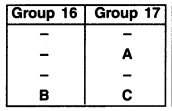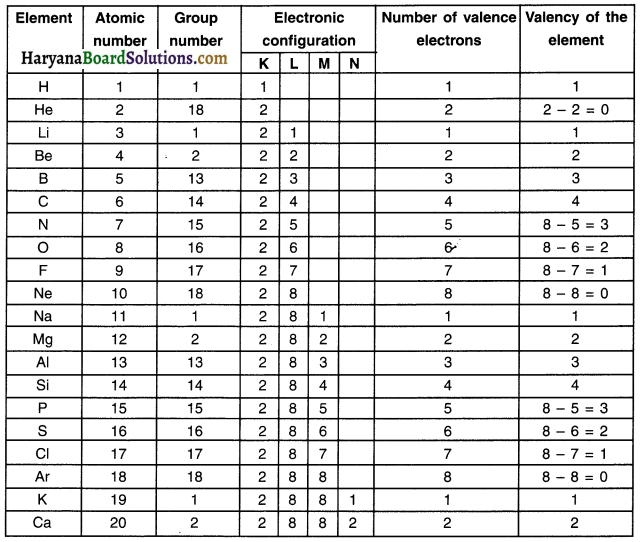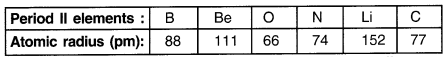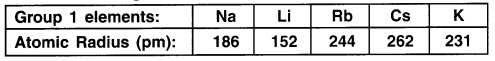# HBSE 10th Class Science Solutions Chapter 5 Periodic Classification of Elements

Haryana State Board HBSE 10th Class Science Solutions Chapter 5 Periodic Classification of Elements Textbook Exercise Questions and Answers.

## Haryana Board 10th Class Science Solutions Chapter 5 Periodic Classification of Elements

HBSE 10th Class Science Periodic Classification of Elements Textbook Questions and Answers

Question 1.
Which of the following statements Is not a correct statement about the trends when going from left to right across the periods of periodic Table?
(a) The elements become less metallic in nature
(b) The number of valence electrons increases
(c) The atoms lose their electrons more easily
(d) The oxides become more acidic
(c) The atoms lose their electrons more easilyQuestion 2.
Element X forms a chloride with the formula XCI2, which is a solid with a high melting point. X would most likely be in the same group of the Periodic Table as ……….
(a) Na
(b) Mg
(c) Al
(d) Si
(b) Mg

Question 3.
Which element has —
(a) two shells, both of which are completely filled with electrons?
(b) the electronic configuration 2, 8, 2?
(c) a total of three shells, with four electrons in its valence shell?
(d) a total of two shells, with three electrons in its valence shell?
(e) twice as many electrons in Its second shell as in Its first shell?
(a) Neon Ne (2, 8)
(b) Magnesum Mg (2, 8, 2)
(c) Silicon Si (2, 8, 4)
(d) Boron B (2, 3)
(e) Carbon C (2, 4)

Question 4.
(a) What property do all elements in the same column of the Periodic Table as boron have In common?
(b) What property do all elements In the same column of the Periodic Table as fluorine have in common?
(a) In Modern Periodic Table, element boron (B) lies in group 13 arid its valency is 3. So, all the other elements belonging to this group will also have valency 3.

(b) In the Modern periodic table, element fluorine (F) lies in group 17. All the elements of group 17 have 7 electrons in their valence shell. Hence, all the other elements belonging to the group of fluorine have valency 1. So, their valency is 1.Question 5.
An atom has electronic configuration 2, 8, and 7.
(a) What is the atomic number of this element?
(b) To which of the following elements would It be chemically similar?
(Atomic numbers are given in parentheses.)
N(7)   F(9)   P(15)  Ar(18)
(a) The atomic number of the element having electronic configuration 2, 8, 7 is 2 + 8 + 7 = 17.

(b) It will be similar to F(9) because electronic configuration of F(9) is 2, 7 i.e. similar to the element having atomic number 17 having 7 valence electrons.

Question 6.
The position of three elements A, B and C In the Periodic Table are shown below –(a) State whether A is a metal or non-metal.
(b) State whether C Is more reactive or less reactive than A.
(c) Will C be larger or smaller in size than B?
(d) Which type of ion, cation or anion, will be formed by element A?
(a) Element A is non-metal.
Element is in 17th group. So, there are 7 electrons in its outermost shell or say the valency is 1.
It will acquire 1 electron to complete the octet. Such a property is shown by non-metals.(b) C is less reactive than A.
Element C is placed below element A. As one goes down in a group, the atomic size of elements increases and hence their reactivity decrease.

(c) C is smaller than B.
Elements C and B belong to same period. As one moves from left to right in a period, the atomic size i.e. the volume decreases. Hence, atomic size of element C will be smaller than B.

(d) Element A will form anion.
Element A has 7 electrons in its valence shell. So, it will tend to gain 1 electron to complete its octet and hence form anion.
A + e → A

Question 7.
Nitrogen (atomic number 7) and phosphorus (atomic number 15) belong to group 15 of the Periodic Table. Write the electronic configuration of these two elements. Which of these will be more electronegative? Why?

 Element Atomic number Electronic configuration Nitrogen (N) Phosphorus (P) 7 15 2, 5, – 2, 8, 5

As one moves down in a group, the electronegativity decreases. Nitrogen’s atomic number is 7. So, It lies above phosphorus. Hence, nitrogen will be more electronegative.

Question 8.
How does the electronic configuration of an atom relate to its position In the Modern Periodic Table?
1. Through electronic configuration we can know the position of the element in the Modern Periodic Table.
2. Through the electronic configuration we can know the number of valence electrons, The valence electrons enables identifying the position of the element in the table.

Example:

• Sodium (Na) has atomic number 11. So, its electronic configuration is 2, 8, 1 (2 + 8 + 1 = 11).
• There is 1 valence electron in this configuration. Hence, sodium (Na) belongs to group 1.
• There are three shells in this configuration. So, sodium lies in 3rd period.Question 9.
In the Modern Periodic Table, calcium (atomic number 20) is surrounded by elements with atomic numbers 12, 19,21 and 38. Which of these have physical and chemical properties resembling calcium?

 Element Atomic number Electronic configuration Calcium Magnesium Strontium 20 12 38 2, 8, 8, 2, — 2, 8, 2, —, — 2, 8, 18, 8, 2

As can be seen in the table above out of the given atomic numbers, only magnesium (Z=12) and strontium (Z=38) have same valence electrons i.e. ‘2’ Hence, these two elements have physical and chemical properties similar to calcium.

Question 10.
Compare and contrast the arrangement of elements in Mendeleev’s Periodic Table and the Modem Periodic Table.

 Mendeleev Periodic Table Modern Periodic Table 1. Elements in this table were arranged on the basis of increasing atomic mass. 2. It contains 7 periods and 8 groups. 3. Mendeleev placed the transition elements arbitrarily in group VIII. 4. In Mendeleev’s table periodicity of elements cannot be explained. 1. Elements in this table were arranged on the basis of increasing atomic number. 2. If contains 7 periods and 18 groups. 3. Transition elements are placed properly in a separate group. 4. Periodicity of elements can be properly explained.

HBSE 10th Class Science Periodic Classification of Elements InText Activity Questions and Answers

Textbook Page no – 81

Question 1.
Did Dobereiner’s triads also exist In the columns of Newlands’ Octaves? Compare and find out.
Yes, Dobereiner’s triads exist even in the columns of Newlands’ Octaves.
Example:

• Lithium (Li), Sodium (Na) and potassium (K) constitute Dobereiner’s triads.
• If we consider Li as the 1st element then the 8th element from it is Na.
• Then, when we consider Na as the l element, then the 8th element from it is K.
• Similarly, Dobereiner’s triad consisting of the elements beryllium (Be), magnesium (Mg) and calcium (Ca) is also included in the column of Newlands’ octaves.
• Thus, Dobereiner’s triads are included in the columns of Newland’s Octaves.

Question 2.
What were the limitations of Doberelner’s classification?
Limitation of Dobereiner’s classification :

• Under Dobereiner’s classification, overall only a limited number of elements could be classified into triads.
• After arranging the elements in triads, it was found that there were certain other elements which could not be classified by Dobereiner’s method.

Question 3.
What were the limitations of Newlands’ Law of Octaves?
Limitation of Newlands’ Law of Octaves:
1. The law of octaves was applicable only upto calcium. After calcium, every 8 element did not possess properties similar to that of let.

2. Newlands thought that there were only 56 elements in nature. He also thought that no more elements would be discovered in the future. However, later, several new elements were discovered that could not be arranged in the table as per Newlands’ law.

3. In order to fit elements any how into his table. Newlands adjusted two elements in the slot even if the properties of elements did not match with other elements.Example:
1. He placed Cobalt (Co) and nickel (Ni) in the same slot and placed these elements in the group of fluorine, chlorine and bromine. But, the properties of these three elements are quite different than cobalt and nickel.
2. Owing to these limitations we can say that Newslands Law of Octaves worked well only with lighter elements.

Textbook Page no – 85

Question 1.
Use Mendeleev’s Periodic Table to predict the formulae for the oxides of the following elements:
K, C, Al, Sl, Ba
1. Valency of oxygen (O) is 2. We can find the valencies of other elements by looking at Mendeleev’s Table.
2. The formulas of oxides with asked elements are given in the table below.

 Element Valency Molecular formula of oxides Potassium (K) Carbon (C) Aluminium (Al) Silicon (Si) Barium (Ba) 1 4 3 4 2 K2O C2O44 OR CO2 Al2O3 Si2O4 OR SiO2 Ba2O2 OR BaO

Question 2.
Besides gallium,which other elements have since been discovered that were left by Mendeleev in his Periodic Table?(any two)
Besides gallium, germanium and scandium have been discovered.

Question 3.
What were the criteria used by Mendeleev in creating his Periodic Table?
Mendeleev used the following criteria for placing elements in his Periodic Table:

Criteria used by Mendeleev for developing periodic table:

• The properties of elements are the periodic function of their atomic masses. Hence, arranging elements in the increasing order of their atomic masses.
• Elements with similar properties are arranged in the same group.
• The formula of oxides and hydrides formed by an element.

Question 4.
Why do you think the noble gases are placed in a separate group?
1. All noble gases are inactive i.e. very inert because they have a complete octet.
2. They do not react with other elements because they are very stable.
3. All of them show similar properties.
4. Owing to all these reasons, noble gases are placed in a separate group.

Textbook Page no – 90

Question 1.
How could the Modern Periodic Table remove various anomalies of Mendeleev’s Periodic Table?
The Modem Periodic Table removed the anomalies of Mendeleev’s Periodic Table as follows:

(1) Position of isotopes:
Isotopes of an element have same atomic number and so their placement was an issue in Mendeleev table. In Modern Periodic Table all the elements were placed in one group to resolve the issue.

(2) Improper position of some pairs of elements. Mendeleev had arranged the elements in the increasing order of their atomic masses. This posed some problems. However, this problem got solved when the elements were placed in increasing order of the atomic number.

(3) Uncertainty of the number of elements that could be discovered.
Elements in Mendeleev’s Periodic Table were placed on the basis of increasing atomic mass. However, the atomic mass does not increase in a specific manner as one moves from one element to another. Hence, one could not predict the number of elements that could be discovered between two elements.

Question 2.
Name two elements you would expect to show chemical reactions similar to magnesium. What Is the basis for your choice?
Elements: Beryllium (Be), Calcium (Ca), etc.
Reason: Magnesium (Mg) has 2 electrons in the valence shell. Hence, all elements such as the two stated above having 2 electrons in the valence shell will belong to the group of magnesium and hence show similar chemical properties. In other words, all elements belonging to same group as that of magnesium will show similar chemical properties.Question 3.
Name —
(a) Three elements that have a single electron in their outermost shells.
(b) Two elements that have two electrons In their outermost shells.
(c) Three elements with filled outermost shells.
(a) Lithium (Li) (Z = 3), Sodium (Na) (Z = 11) and Potassium (K) (Z = 19) have 1 electron in their last orbits.
(b) Magnesium (Mg) (Z =12) and Calcium (Ca) (Z = 20) have 2 electrons In their last orbits.
(c) Noble gases namely Helium (He), Neon (Ne). Argon (Ar), etc. have their outermost shell completely filled with electrons.

Question 4.
(a) Lithium, sodium, potassium are all metals that react with water to liberate hydrogen gas. Is there any similarity between the atoms of these elements?
(b) Helium is an unreactive gas and neon is a gas of extremely low reactivity. What, if anything,
do their atoms have In common?
(a) Similarities between atoms of lithIum (LI), Sodium (Na) and potassium (K):

• All these elements are highly reactive.
• They have same valence electrons i.e. 1.
• They readily loose electrons and become positive ions.

(b) Similarity between helium and neon:

• Both are noble gases.
• Both belong to the same group.
• Outer shell of both is completely filled.

Question 5.
In the Modern Periodic Table, which are the metals among the first ten elements?
Among the first 10 elements, only 2 elements namely lithium (Li) and beryllium (Be) are metals.

Question 6.
By considering their position in the Periodic Table, which one of the following elements would you expect to have maximum metallic characteristic? Ga, Ge, As, Se and Be
1. Element (Be) belongs to 2nd group and (Ga) belongs to 13th. All other elements belong to higher groups.
2. As one moves from left to right i.e. in the increasing order of the groups, the metallic character of an element decreases.
3. Hence, elements (Be) and (Ga) will show maximum metallic characteristic.

Activities

Activity 1.

Looking at its resemblance to alkali metals and the halogen family, try to assign hydrogen a correct position In Mendeleev’s Periodic Table.

Question 1.
To which group and period should hydrogen be assigned?
Hydrogen has the lowest atomic number i.e. 1. Its position is quite controversial and so it is difficult to assign hydrogen a fixed location. However, we can put it at group 1. period 1 position.

Activity 2.

Consider the isotopes of chlorine, CI-35 and Cl-37.

Question 1.
Would you place them in different slots because their atomic masses are different? Or would you place them In the same position because their chemical properties are the same?
No the two isotopes cannot fit at two different positions. Moreover, chemical properties of both are similar and so they should be placed at the same position in group 17.Activity 3.

Question 1.
How were the positions of cobalt and nickel resolved in the Modern Periodic Table?
In Modern Periodic Table the elements were arranged in increasing order of their atomic number. So, cobalt with atomic number 27 was placed in group 9 whereas nickel having atomic number 28 was placed in group lo.

Question 2.
How were the positions of isotopes of various elements decided in the Modern Periodic Table?
In the Modern Periodic Table, all the isotopes of an element were placed at a same location.

Question 3.
Is it possible to have an element with atomic number 1.5 placed between hydrogen and helium?
The atomic number of an element to be represented in the Modern Periodic Table must be a whole number. Since 1.5 is a fractional number the element cannot be placed in the table.

Question 4.
Where do you think should hydrogen be placed in the Modern Periodic Table?
Atomic number of hydrogen is 1 and so it should be placed at group 1 and period 1 position in the Modern Periodic Table.

Activity 4.

Question 1.
Look at the group 1 of the Modern Periodic Table, and name the elements present in it.
Hydrogen (H), Lithium (Li), Sodium (Na), Potassium (K), Rubidium (Rb), Cesium (Cs)

Question 2.
Write down the electronic configuration of the first three elements of group 1.
H(K=1), Li(K=2,L=1), Na(K=2,L=8,M=i)

Question 3.
What similarity do you find In their electronic configurations?
All elements contain one electron in their outermost shell.

Question 4.
How many valence electrons are present in these three elements?
The three elements have one electron in their respective valence shell.

Activity 5.

Question 1.
If you look at the Modern Periodic Table, you will find that the elements Li, Be, B, C, N, O, F, and Ne are present In the second period. Write down their electronic configurations.
Li (2, 1), Be (2, 2), B (2, 3), C (2, 4), N (2, 5), 0(2, 6), F(2, 7), Ne (2, 8)

Question 2.
Do these elements also contain the same number of valence electrons?
These elements contain different number of valence electrons.

Question 3.
Do they contain the same number of shells?
Yes, all these elements contain the same number of shells, i.e. two. The shells are K and L.Activity 6.

Question 1.
How do you calculate the valency of an element from its electronic configuration?
The valency of an element is determined by the number of valence electrons present in the outermost shell of an atom.
For elements of group 1, 2, 13 and 13, the valency is equal to the number of valence electrons.
For elements of group 15, 16, 17 and 18, the valency is equal to 8 minus the number of valence electrons.

Question 2.
What is the valency of magnesium with atomic number 12 and sulphur with atomic number 16?
Atomic number of Mg is 12. So, its electronic configuration is 2, 8, 2.
Hence, valency of Mg is 2.
Atomic number of S is 16. So its electronic configuration is 2, 8. 6.
Hence, valency of S = 8 – 6 = 2.

Question 3.
Similarly, find out the valencies of the first twenty elements.Question 4.
How does the valency vary In a period on going from left to right?
As you move in the period from left to right, the valency first increases from 1 to 4. Then it decreases from 4 to O.

Question 5.
How does the valency vary In going down a group?
Valency does not change within a group.Activity 7.

Question 1.
Atomic radii of the elements of the second period are given below:Question 2.
Arrange them In decreasing order of their atomic radii.
Li > Be > B > C > N > O.

Question 3.
Are the elements now arranged in the pattern of a period in the Periodic Table?
Yes

Question 4.
Which elements have the largest and the smallest atoms?
Largest atoms = Lithium; Smallest atoms = Oxygen

Question 5.
How does the atomic radius change as you go from left to right in a period?
Atomic radius decreases as we go from left to right in a period.

Activity 8.

Question 1.
Study the variation in the atomic radii of first group elements given below and arrange them in an increasing order.Li < Na < K < Rb < Cs

Question 2.
Name the elements which have the smallest and the largest atoms.
Largest atoms = Cesium; Smallest atoms = Lithium

Question 3.
How does the atomic size vary as you go down a group?
Atomic size increases as we go down in a group

Activity 9.

Question 1.
Examine elements of the third period and classify them as metals and non-metals.
Sodium, magnesium and aluminium are metals, silicon is non-metal (metalloid) and Phosphorus, sulphur, chlorine and argon are non-metals.

Question 2.
On which side of the Periodic Table do you find the metals?
Metals are found on the left hand side of the Periodic table.

Question 3.
On which side of the Periodic Table do you find the non-metals?
Non-metals are found on the right hand side of the Periodic Table.Activity 10.

Question 1.
How do you think the tendency to lose electrons changes In a group?
As we move from top to bottom in a group, the tendency to lose electrons increases

Question 2.
How will this tendency change in a period?